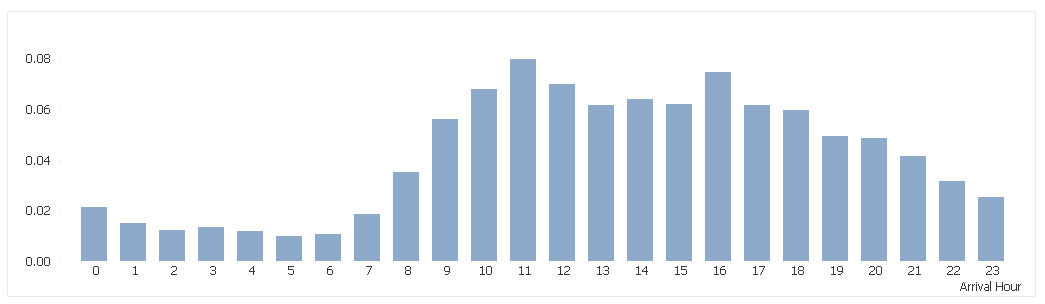# QlikView App Development

Discussion Board for collaboration related to QlikView App Development.

HighlightedNot applicable

## Average....Strange this happening

Hi

I have a chart showing the total arrivals by hour of the day over the last two weeks:

Dimension = [ArrivalHour]

Expression = sum( {<.Last2Weeks={'1'}>}total_arrivals)I need to change this to show the average arrivals per hour for the whole two week period.

However, when I change the expresion to this :

sum( {<.Last2Weeks={'1'}>}total_arrivals) / sum( total {<.Last2Weeks={'1'}>}total_arrivals)

the chart is showing less than zero per hour for the two week period :Can anyone explain why this is happening ?  The average for 12 midday should be 33.

Many thanks for any help!

TJM

Tags (1)
3 RepliesMVP

## Re: Average....Strange this happening

Not sure I understand your expected output. May be this:

Avg( Total {<.Last2Weeks={'1'}>}total_arrivals)MVP

## Re: Average....Strange this happening

If you divide the absolute number per bin by the absolute total number, you get the percentage of that bin compared to the total.

In your case, approx. 6-7% of the arrivals happen around 12 midday.

An average arrival could be calculated by

sum( {<.Last2Weeks={'1'}>}total_arrivals) / Count( DISTINCT {<.Last2Weeks={'1'}>}  DateFIELD)

or

sum( {<.Last2Weeks={'1'}>}total_arrivals) / 14   /* 14 days in period */MVP

## Re: Average....Strange this happening

Right now what you are doing is converting your total_arrival data into percentage.... basically if across the 24 hours in the day for 2 weeks you have a sum of 2000 (for example) and 0 hour is 50, then you are doing 50/2000.

Have you tried Avg() instead of Sum?

Avg({<.Last2Weeks={'1'}>} total_arrivals)

or

Sum({<.Last2Weeks={'1'}>} total_arrivals)/14